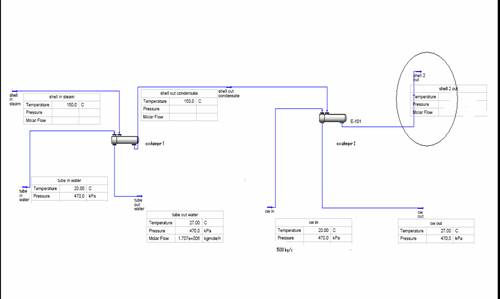Courses

1. CHE 590 – Special Topics in Polymer Reaction Engineering

2. CHE 543 – Polymeric Materials

1. CHE 300 – Transport Phenomena II ( Heat Transfer)

2. CHE 304 – Transport Phenomena III ( Mass Transfer)

3. CHE 306 - Stagewise Operations

4. CHE 325 – Chemical Engineering Computing Laboratory
5. CHE 463 – Polymer Technology

6. CHE 402 -

Short Courses:

1. Polymer Reaction Engineering, April 2008 - Coordinated
2. Polymer degradation and stabilization: Emphasis on Polymer Additives, November, 2008 – Coordinated

Course Books

Educational Demos and Examples

Demos Using Mathematica

Examples Using Hysis (Design of Heat Exchangers)

Problem 1

A feed water heater uses a shell and tube heat exchanger with condensing steam in one shell pass at 120°C. Water enters the tube at 30°C and makes four passes. The UA is 15720 W/C. For 2.5 kg/s mass flow of water

a)     calculate the exit water temperature

b)    heat duty of the heat exchanger

c)     Flow rate of the steam required

d)    Plot Heat flow vs. Temperature for shell and tube side flow

Solution Problem 1 Using Hysis

Problem 2

Hot water at 90°C and 1 atm is used in tubes of a finned tube shell and tube heat exchanger. Air flows across the fins and enters at 1 atm, 30°C and leaves at 45°C (1 atm ) at the same pressure. The value of UA is 415 W/C . if the exit water temperature is 65°C calculate

a)     The flow rate of air required

b)    The heat exchanger duty

c)     The flow rate of water

d)    Plot Heat flow vs. Temperature for shell and tube side flow

e)     Solve the problem by taking tube side pressure drop is 10 kpa

Assume 1 shell pass and 2 tube passes

Solution Problem 2 Using Hysis

Problem 3

Hot air from furnace at 120°C is entering shell side of shell and tube heat exchanger at a pressure of 1 atm and leaving at 30°C. Cooling water at 20°C and I atm enters the tube side at a flow of 2500 kg /hr making two tube passes. If the heat duty is 200 KW, calculate

a)     The exit water temperature

b)    The air flow rate

c)     The value of UA

d)    The value of UA when the number of tubes is changed to 200

Assume the shell side pressure drop 10 kPa and Tube side pressure drop as 15 kPa.

Solution Problem 3 Using Hysis

Problem 4

A shell and tube heat exchanger has condensing steam at 100°C in the shell side with one shell pass. Two tube passes are used with the air in the tubes entering at 10°C 1 atm and flow rate of 15000 kg /hr. if the value of UA is 4500

a)     Calculate the exit air temperature

b)    The duty of heat exchanger

c)     The flow rate of air

d)    The Log mean temperature difference (LMTD)

e)     Solve the problem first by assuming pressure drop as zero for shell and tube side and then 25 kPa for both shell and tube side.

Solution Problem 4 Using Hysis

Problem 5

A steam condenser for a large power plant has steam condensing at 38°C on the shell side of a shell and tube heat exchanger. Water serves as the coolant on the tube side, entering at 20°C (50 kPa) and leaving at 27°C . The exchanger involves one shell pass and two tube passes. If the heat duty is 700 MW , calculate

a)     Value of UA if the number of tubes in the shell is 300

b)    Water flow rate required

c)     Solve the problem by assuming shell side and tube side pressure drop as zero and then by assuming only tube side pressure drop as 5 kPa.

Solution Problem 5 Using Hysis

Problem 6

A steam condenser for a large power plant has steam condensing at 150°C on the shell side of a shell and tube heat exchanger. Water serves as the coolant on the tube side, entering at 20°C (470 kPa) and leaving at 27°C . The exchanger involves one shell pass and two tube passes. If the heat duty is 250 MW , Assuming zero pressure drop for both shell and tube side calculate

a)     Water flow rate required

b)    Steam flow rate required

Connect another similar shell and tube heat exchanger with the shell inlet same as shell outlet of the first heat exchanger i.e condensate at    150°C leaving the first heat exchanger. Cooling water enter the tube side at 20°C (470kPa) and leaving at 27°C  (470 kPa). If the shell side pressure drop is zero and the cooling water flow rate is 1700 kg/s calculate the shell side exit temperature and heat duty of the second heat exchanger.

The diagram is given asSolution Problem 6 Using Hysis

Problem 7

A single shell and tube heat exchanger with two tube passes is used to condense steam at 100°C (1 atm) on the shell side. Water is used on the tube side and enters the exchanger at 20°C (1 atm ) with a flow rate of 1.0 kg/s. the value of UA is 2000 W/C. Assuming the tube side pressure drop as 10 kPa and shell side pressure drop as 0 kPa, calculate

a)     T exit water temperature

b)    The flow rate of steam through the shell

c)     The heat duty of the exchanger

d)    The log mean temperature difference (LMTD)

Solution Problem 7 Using Hysis